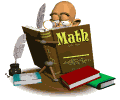• 5th Grade Math:This year in math, we will be using the Mcgraw Hill My Math textbook to supplement. This textbook also has a online edition that can be accessed through https://connected.mcgraw-hill.com/connected/login.doIn each students math binder, there will be a label inside with the childs username and password information.

1. Number Sense - In this unit, students will learn how to express, order, and compare whole numbers up to the billions and decimal numerals up to the hundred-thousandths. Students will round whole numbers to specific place values and explain the value of a digit based on its position in a number
.
2. Multiplication - Students will learn how to multiply multi-digit whole numbers using various strategies and apply their understanding to solve word problems.
3. Division - Students will use various strategies to divide whole numbers and apply their understanding to solve word problems and aid in mental math calculations. Now that all four operations have been taught, students will apply the fundamental order of operations.

4. Fractions - Students will develop a conceptual understanding of how to add, subtract, multiply, and divide fractions and mixed numbers. This knowledge will be applied to real word scenarios.

5. Decimals - Students will develip a conceptual understanding of how to add, subtract, multiply, and divide decimal numerals. This knowledge will be applied to real word scenarios.

6. Geometry and Measurment - Students will learn how to convert and develop a standard reference of measurements in both U.S. Customary and Metric systems. Students will learn how to classify polygons and 3-dimensional solids.

7. Patterns and Algebra - Students will learn how to analyze patterns and relationshups, as well as create and evaluate numerical expressions.

8. Data Analysis - Students will learn how to represent and interpret data using a variety of graphic representations.